# Design of ESP Notes | Study Environmental Engineering - Civil Engineering (CE)

## Civil Engineering (CE): Design of ESP Notes | Study Environmental Engineering - Civil Engineering (CE)

The document Design of ESP Notes | Study Environmental Engineering - Civil Engineering (CE) is a part of the Civil Engineering (CE) Course Environmental Engineering.
All you need of Civil Engineering (CE) at this link: Civil Engineering (CE)

DESIGN OF ELECTROSTATIC PRECIPITATOR

Introduction

An electrostatic precipitator (ESP) is a particle control device that uses electrical forces to move the particles out of the flowing gas stream and onto collector plates. The particles are given an electrical charge by forcing them to pass through a corona, a region in which gaseous ions flow.

INFORMATION REQUIRED FOR DESIGNING OF ESP

The efficiency of an ESP depends upon two factors

• The size of the unit i.e. total square ft. of the collecting plate area
• Amount of independent electrical energisation

In addition following details are required for designing an ESP

1. Source of the emission : Properties of the process by which the pollutants are produced

2. Particle size distribution

3. Chemical analysis of dust in relation to particle size

4. Specific eclectic resistivity of dust

5. Dust concentration of clean gas

6. Required dust concentration of clean gas(efficiency)

7. Properties of gas: composition, temperature, pressure.

8. Corrosive properties of gas

9. Gas flow rate

Apart from these variables the design of ESP also include the determination of ancillary factors such as rappers to shake the dust loose from the plates, automatic control system, measures for ensuring high-quality gas flow, dust removal system, provisions for structural and heat insulation and performance monitoring system.

Firstly size distribution of dust is determined; from the information of size distribution of dust the migration velocity is calculated. After that number of charge on a particle is calculated by using appropriate equation. On the basis of precipitation rate the collecting surface area for a given efficiency at a particular flow rate is calculated by using Deutsch-Andersen relationship.

PARTICLE CHARGING

According to kinetics, the electron energy Qof an originally neutral dust particle is given by: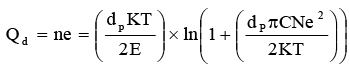(2.6.1)
Where, N=number of electronic charges; dp=dust particle dia.; K=1.38*10-23(J/K); T=Absolute Temp.; E=1.602×10-19 [C]–electrons; N=free ion density; C=(2kT/mi)1/2 Charging velocity is very fast at the beginning but slow down with time.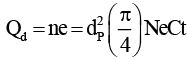(2.6.2)

Field Charging: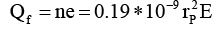(2.6.3)
Where, n=Number of electronic charge; rp=Radius of particle; E=Average field intensity, E=1.602*10-19

MIGRATION VELOCITY

The velocity of charged particle suspended in a gas under the influence of an electric field is known as migration velocity. The particle migration velocity is the most important parameter and is function of a large number of operation quantities such as- Electric field strength, particle size, gas viscosity, properties of the dust. Principal forces acting on particle are gravitational force, electric force, viscous force and inertial force.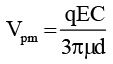(2.6.4)
Where, Vpm=Particle migration velocity towards the collector electrode; q=ne, value of n depends upon types on charging (diffusion or field); C=Cunningham correction factor; E=Collector electric field; m=gas viscosity; and d=particle size (µm).

Table 2.6.1 Effective migration velocity (m/s) for various type of dusts.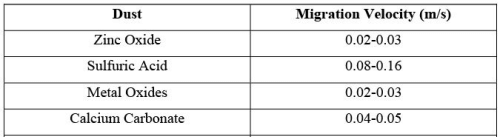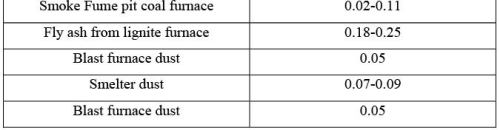COLLECTION EFFICIENCY OF ESP

The collection efficiency of an ESP as a function of gas flow rate and precipitator size is given by the Deutsch-Andersen Equation

Assumptions:

a. Repulsion effect is neglected.
b. Uniform gas velocity throughout the cross section
c. Particles are fully charged by field charging.
d. No hindered settling effect. Collection efficiency mathematically expressed as follows: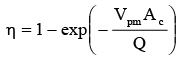(2.6.5)
η=Fractional Collection Efficiency; Ac=Area of the collection electrode; Vpm=Particle migration velocity; Q=Av=Volumetric flow rate of gas; v=gas velocity.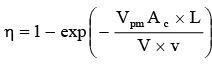(2.6.6)
Where, V=Volume of precipitator. For cylindrical type collector: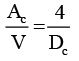(2.6.7)
For parallel plate: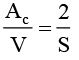(2.6.8)
Where, S=distance between the two parallel plates; V=Gas Volume; W=Precipitation Rate Parameter; A=Plate Area; Q=Volumetric Flow Rate of Gas.
When the charged particle passing a charge qp is in a region where an electric field strength of Ec is present, a force F will act on particle.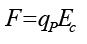(2.6.9)

The migration of particle towards the collector is resisted by a drag force and the net force on the particle is zero when it moves with a constant drift velocity (vpm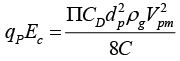(2.6.10 )
For small particle stokes law is applicable.  Hence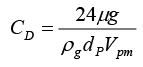( 2.6.11)
Substitute CD in above equation it gives vpm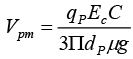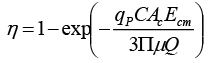(2.6.12 )
Where, qp=Particle charge; Ac=collector surface area; Ecm=electric field strength; d=particle size; µ=gas viscosity

C = Cunningham correction factor =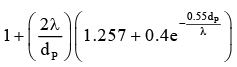(2.6.13)

For standard air, λ=0.066µm
For standard air, above equation becomes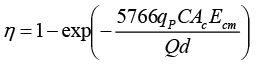(2.6.14 )
Collection efficiency of different types of precipitators

Ψ=(Mp1-Mp2)/Mp1.          (2.6.15)

Where, Mp1 and Mp2 being the mass of dust per unit volume of the gas stream at the entrance and exit of the precipitator. The volumetric flow rate Vg in a precipitator by the equation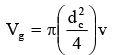(2.6.16 )
For a plate precipitators
Vg =  nahv         (2.6.17)

Where; h=Height of the plate which is equal to the height of the channels

The equation is related to gas velocity v and number n of the element for pipe precipitators. If particles are of a solid, the collected particles are removed from electrode by shaking it in a process known as rapping. If particles are of a liquid, after collecting on the electrode the liquid then flows down the electrode by action of gravity and collects at the bottom. The particle charging process is done by means of corona surrounding a highly charged electrode, such as wire .

DUST RESISTIVITY

• Dust resistivity is the most important dust property.
• With high dust resistant a large voltage in the dust layer is observed accumulated by a decreasing current.
• Specific Dust Resistivity: is the resistivity of a layer of dust will a layer thickness of 1 cm over a collection area of 1m2
• The Specific dust resistivity is designed the symbol ρrs and measured in Ωcm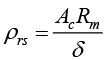(2.6.18)
Where, Ac=collection area; Rm=mean electric resistivity; δ=dust layer thickness

Table 2.6.1. Ranges for ESP design parameters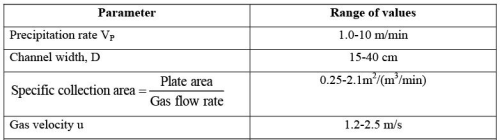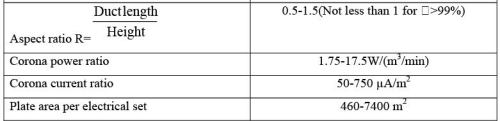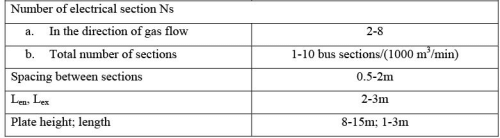Problem 2.6.1: A plate type ESP use in a cement plant for removing dust particles consist of 10 equal channels. The spacing between plates is 15 cm and the plates are 3 m high and 3 m long. Unit handles 20,000 m3/h of gas.
a) What is the efficiency of collection plates?
b) What is the collection rate of particles having density 9.2 gm/m3?
c) What should be the length of the plate for achieving efficiency of 99% keeping other parameter same?

Solution: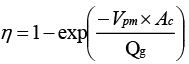Ac = 2 x n x h x l

q = η x Qg x ρ

Given: Particle migration velocity (Vpm)=0.1 m/sec; Number of plates (n)=10; Height of plate (h)=3 m; Length of plate (l)=3 m; Gas flow rate (Qg)=20000 m3/h=2.7778 m3/sec; Density of particle (ρ)=9.2 g/m3.
Total area of collection plates (Ac)=2×10×3 m×3 m=180 m2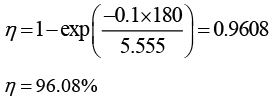The collection rate  q = 0.9608x5.555 x 9.2 = 49.1070g / sec
Let us assume the length of plate is l m.
Therefore, the total collection area of plate (Ac ) becomes 40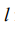m2.
The length of plate can be obtained from following equation: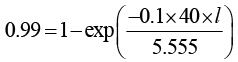l = 95.9314m

a) The efficiency of collection plate is 96.08%.
b) The collection rate of particles having density is 49.1070g/sec.
c) Length of the plate for achieving efficiency of 99% keeping other parameter same is 95.93 m.

Problem 2.6.2: An ESP handles 107 ft3/min of gas. It uses 3.6 Amp current and has 28000 ft2 collection plate areas. At the present operating temperature, the dust resistivity is 3×1011 Ω-cm. It has been suggested that the gas cooled to reduce the dust resistivity to 7×1010 Ω-cm assuming that average dust thickness is 0.45 inch and that voltage difference between the charging walls and outer surface of the dust layer is 30 kV must be maintained in both cases. Estimate the reduction in power requirement that cooling the gas to get neglect the effect of gas temperature on charging and drift velocity.

Solution: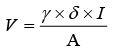P=Vx I

Given: Gas flow rate (Qg)=107 ft3/min; Current (I)=3.6 A; Total area of collection plates (Ac)=28000 ft2=26.01×106 cm2; Dust resistivity (γ1)=3×1011 Ω-cm; Dust resistivity (γ2)=7×1010 Ω-cm; Dust thickness (δ=0.45 inch=1.143 cm. Assuming that V1 is the voltage when dust resistivity is γ1 (kV) and voltage is V2 when dust resistivity is γ2 (kV) and P is the power input (kW)

For a dust resistivity of 3×1011 Ω-cm,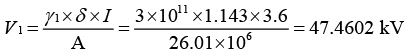Total applied voltage is 30 kV+47.4602 kV=77.4602 kV Therefore, the power input when dust resistivity is γ1
P=V1I= 77.4602´3.6 = 278.856 kW

For a dust resistivity of 5×1010 Ω-cm,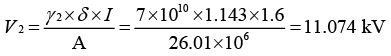Total applied voltage is 30 kV+11.07 kV=41.074 kV The power input P2 =V2I= 41.074x3.6 = 147.886 kW
Therefore by cooling of gas, power input gets reduced to ΔP=P1- P2.
ΔP=278.856-147.886=130.97 kW

The document Design of ESP Notes | Study Environmental Engineering - Civil Engineering (CE) is a part of the Civil Engineering (CE) Course Environmental Engineering.
All you need of Civil Engineering (CE) at this link: Civil Engineering (CE)Use Code STAYHOME200 and get INR 200 additional OFF

## Environmental Engineering

14 videos|74 docs|70 tests

Track your progress, build streaks, highlight & save important lessons and more!

,

,

,

,

,

,

,

,

,

,

,

,

,

,

,

,

,

,

,

,

,

;